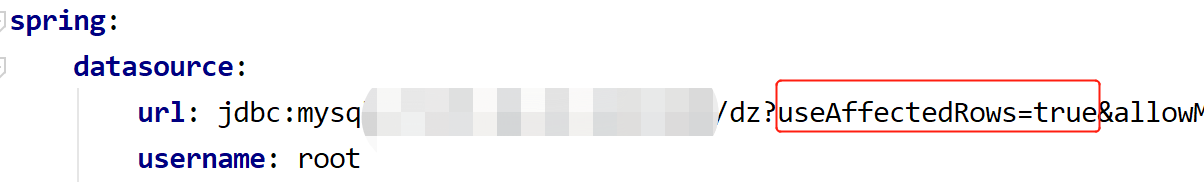# [Solved] The number of rows returned by the mybatis UPDATE statement is always 1 or useaffectedrows = true

The number of rows returned by the mybatis UPDATE statement is always 1 or useaffectedrows = true

Problem solving explanation useaffectedrows = true

solve the problem

``````     This is because the database url connection is missing useAffectedRows=true
```````````` The problem is solved here, but I'll explain it later useAffectedRows=true.
``````

Explain useaffectedrows = true

`````` useAffectedRows defaults to false, and the value returned is the number of rows matched.
useAffectedRows = true, the value returned is the number of rows affected.
``````